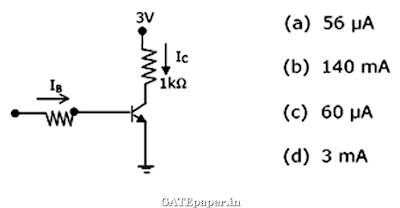### GATE 2004 ECE Video Solutions on EDC (Electronic Devices and Circuits)

1. The impurity commonly used for realizing the base region of a silicon NPN transistor is
a. Gallium
b. Indium
c. Boron
d. Phosphorous

2. If for a silicon NPN transistor, the base to emitter voltage (VBE) is 0.7 volts and collector to base voltage (VCB) is 0.2 volts, then the transistor is operating in the
a. Normal active mode
b. Saturation mode
c. Inverse active mode
d. Cutoff mode

3. Consider the following statements S1 and S2.
S1: the β of a BJT reduces if the base width is increased
S2: the β of a BJT increases if the doping concentration in the base is increased
Which of the following is correct?
a. S1 is FALSE and S2 is TRUE
b. Both S1 and S2 are TRUE
c. Both S1 and S2 are FALSE
d. S1 is TRUE and S2 is FALSE

4. Assuming VCEsat = 0.2 volts and β = 50, the minimum base current (IBmin) required to drive the transistor in figure to saturation is5. The given figure is the voltage transfer characteristic of6. In abrupt PN junction, the doping concentrations on the P side and N side are NA = 9 x 1016 cm-3 and ND = 1 x 1016 cm-3 respectively. The PN junction is reverse biased and the total depletion width is 3 µm. The depletion width on the P side is
a. 2.7 µm
b. 0.3 µm
c. 2.25 µm
d. 0.75 µm

7. The resistivity of a uniformly doped N type silicon sample is 0.5 Ω-cm. If the electron mobility (µn) is 1250 cm2/V-sec and the charge of an electron is 1.6 x 10-19 coulomb, then the donor impurity concentration (ND) in the sample is
a. 2 x 1016 / cm3
b. 1 x 1016 / cm3
c. 2.5 x 1015 / cm3
d. 2 x 1015 / cm3

8. Consider an abrupt PN junction. Let Vbi be the built in potential of this junction and VR be the applied reverse bias. If the junction capacitance (CJ) is 1 pF for Vbi + VR = 1 volt, then for Vbi + VR = 4 volts, the value of CJ will be
a. 4 pF
b. 2 pF
c. 0.25 pF
d. 0.75 pF

9. Consider the following statements S1 and S2.
S1: the threshold voltage (VT) of a MOS capacitor decreases with increase in gate oxide thickness
S2: the threshold voltage (VT) of a MOS capacitor decreases with increase in substrate doping concentration.
Which of the following is correct?
a. S1 is FALSE and S2 is TRUE
b. Both S1 and S2 are TRUE
c. S1 is TRUE and S2 is FALSE
d. Both S1 and S2 are FALSE

10. The drain of an N channel MOSFET is shorted to the gate so that VGS = VDS. The threshold voltage (VT) of MOSFET is 1 volt. If the drain current (ID) is 1 mA for VGS = 2 volts, then for VGS = 3 volts, ID is
a. 2 mA
b. 3 mA
c. 9 mA
d. 4 mA

11. The longest wavelength that can be absorbed by silicon, which has the band gap of 1.12 eV is 1.1 µm. If the longest wavelength that can be absorbed by another material is 0.87 µm, then the band gap of this material is
a. 1.416 eV
b. 0.886 eV
c. 0.854 eV
d. 0.706 eV

12. The neutral base width of a bipolar transistor biased in the active region is 0.5 µm. The maximum electron concentration and the diffusion constant in the base are 1014 cm-3 and Dn = 25 cm2/sec respectively. Assuming negligible recombination in the base, the collector current density is
a. 800 A/cm2
b. 8 A/cm2
c. 200 A/cm2
d. 2 A/cm2

13. Assuming that the β of the transistor is extremely large and VBE = 0.7 volts, IC and VCE in the circuit shown are14. In the voltage regulator shown, the load current can vary from 100 mA to 500 mA. Assuming that the zener diode is ideal (i.e. the zener knee current is negligibly small and zener resistance is zero in the breakdown region), the value of R is